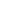# Use number bonds to break apart three-digit minuends and subtract from the hundred

EngageNY 60 min(s)
In this lesson, students extend mental math strategies to subtract tens by using number bonds. They learn to decompose the minuend into 100 and another number. Then they subtract the subtrahend from 100 and add the difference to the other number that results from the decomposition.